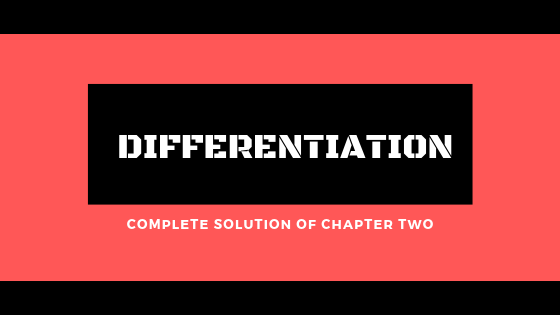## \$type=ticker\$count=12\$cols=4\$cate=0

Are you looking for 2nd-year maths chapter 2 notes with a complete solution?

You have come to the right place.

In this post, you will get the complete solved exercise of second-year math chapter 2.

## What is a Derivative?

The derivative is a way to show rate of change: that is, the amount by which a function is changing at one given point. For functions that act on the real numbers, it is the slope of the tangent line at a point on a graph.

## BENEFITS OF THE NOTES

Why this 2nd-year maths notes of chapter 2 are very important?. The reason behind this is that these notes are written according to your board exam paper point of view. With the help of these notes, you can clear your concepts and also you will be able to solve the objective part of your 2nd-year math paper. So that's why these single notes covered all the aspects of math paper-like long questions, short questions, definitions and MCQs.

You can eaisly get 20 marks in your board exam after preparing this chapter.

Click on the following link to get complete solutions of the 2nd year math notes in PDF.

## FOR WHICH BOARD THE NOTES ARE FOR?

These notes are as per  latest syllabus/course prescribed by Punjab Text Book Board Lahore and FBISE.
These notes are very useful for second-year pre-engineering students as well as for ICS student2nd year math chapter 2 s.FBISE and all the boards of Punjab province have the same course, so it will be very useful to you if you belong to
• Lahore Board
• Gujranwala Board
• Rawalpindi Board
• Sargodha Board
• Multan Board
• Federal Board

### What Is Inside 2nd-Year Maths Chapter 2 PDF?

2nd Year math chapter 2 notes pdf consists of a complete solution of second-year math chapter 2. All exercises of the math chapter 2 solutions with examples. Definitions of 2nd-year math chapter 2 also included in this PDF.

Topics which are included in this pdf are the average rate of change and the derivative of the functions. Find f′(x) from the definition of the derivative, differentiation of expressions of the types. Theorems of differentiation, the chain rule, a derivative of inverse functions.  A derivative of a Function given in the form of parametric Equations. Differentiation of Implicit Relation is also part of this PDF. Derivatives of Trigonometric Function and Derivatives of Inverse Trigonometric Functions. These topics are also included in the 2nd year math chapter 2 pdf which is Derivative of Exponential Functions.erivative of the Logarithmic Function. Logarithmic Differentiation and Derivative of Hyperbolic Function. The derivative of the Inverse Hyperbolic Function and The Series Expansions of Function Tailor Series Expansions of Function are also included. Geometrical Interpretation of a Derivative to Increasing and Decreasing Function and Successive Differentiation ( or Derivatives). The last topic is Relative to Extrema Critical Values and Critical Points.

Here is the complete Solution Of 2nd Year Maths Chapter 2. You Can preview online or you can download easily by click on the following buttons.

#### Note:

• You can also subscribe to ourName

10th Class,2,11th Class,6,12th Class,10,9th Class,17,Fbise,1,Interviews,3,PAPER ATTEMPTING,2,Past Papers,1,successful,2,
ltr
item
Al-Qalam Coaching Center: Free Download 2nd Year Math Chapter 2 Notes In PDF For All Exercises.
Free Download 2nd Year Math Chapter 2 Notes In PDF For All Exercises.